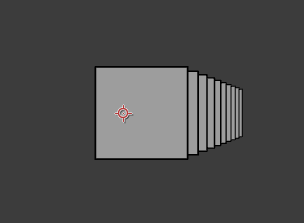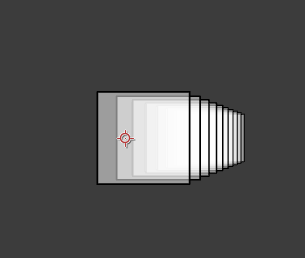# Render transparent objects

Normally, we get the following result when we are about to render a stack of transparent cubes.normalCubes.lua

version3()
in2out()

dim3()
fill(1,.5)

move(width/2, height/2)

for i = 1,10 do
move(20,0,200)
cube()
end


The result was wrong because we cannot see the behind cubes.

We should adjust the render order (farthest to nearest) basing on the camera distance to obtain the right blending color.sortCubes.lua

version3()
in2out()

dim3()
fill(1,.5)

sortarr = {}

beginGroup()
move(width/2, height/2)
for i = 1,10 do
move(20,0,200)
local x,y,z = global2screen(local2global(0,0,0)) -- Get camera distance
sortarr[i] = {z,getTransformMatrix()} -- {distance, transform status} pairs
end
endGroup()

table.sort(
sortarr,
function(a,b) return a > b end -- Sort by z distance
)

for i = 1,10 do
beginGroup(sortarr[i]) -- Apply transform status
cube()
endGroup()
end